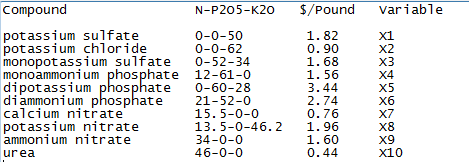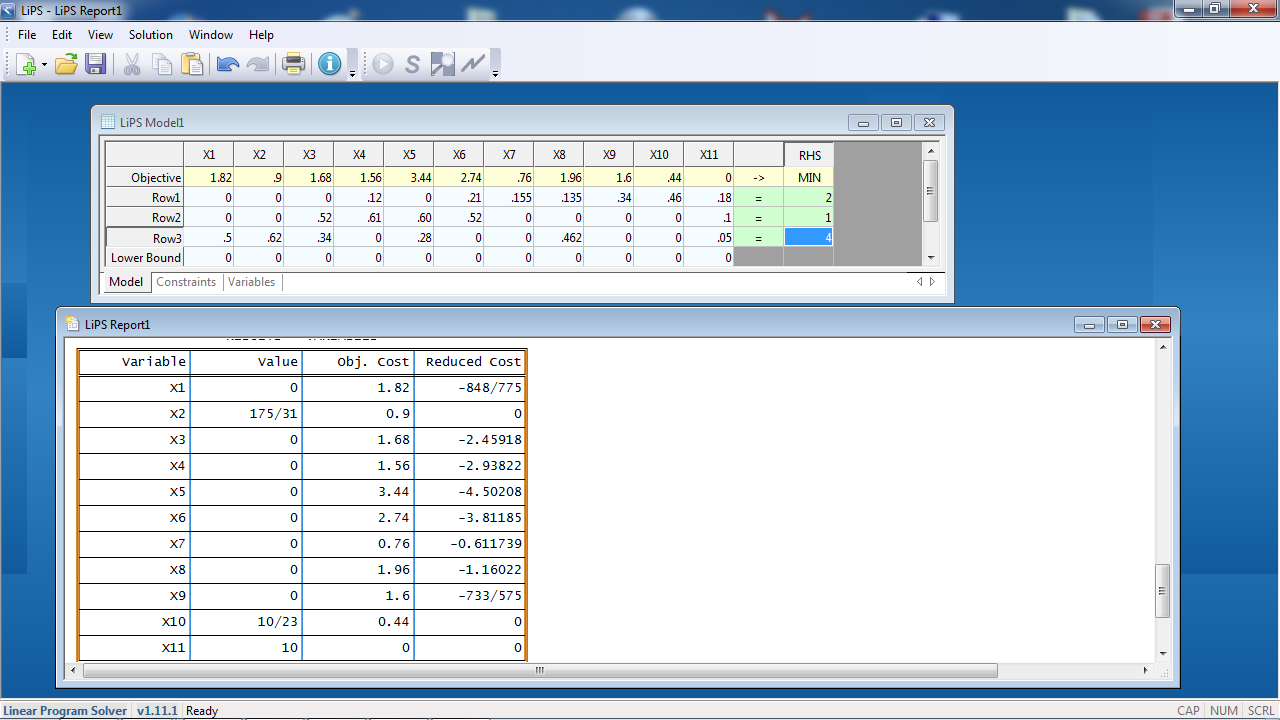View Single Post03-22-2017, 09:35 PM   #4 (permalink)
cincinnana
container grower

Location: Southwest Ohio U.S.A.🇺🇸
Zone: HZ 6/5 Microclimate - Elevation 750 feet- 228.60 meters
Join Date: May 2012
Posts: 8,538
BananaBucks : 703
Feedback: 7 / 100%
Said "Thanks" 3,842 Times
Was Thanked 11,366 Times in 4,746 Posts
Said "Welcome to Bananas" 1,819 TimesRe: FYI, How Professionals Select Fertilizer Ingredients

Quote:
 Originally Posted by aruzinskyProfessionals use Linear Programming (LP) software to select ingredients such that the cost, e.g., \$/lb., is minimized. I will give you a simplified example. First, download this free software: https://sourceforge.net/projects/lipside/ and read my review. Then, you need a list of potential ingredients with costs such as this:And, in addition, suppose you have some 18-10-5 as a possible ingredient that you, otherwise, wouldn't use. You set the cost to 0 or less because it is wasting space. (X11 in the screenshot) Suppose you want to make fertilizer with the ratio 2:1:4. You enter the data into the LP software as follows and calculate the results:In the RHS column, the 2, 1, 4, represent the pounds of N, P2O5, and K20 but we pretend it is N, P, K. In the results, everything that won't be used has the value zero. The values that are nonzero are the pounds of ingredients to be mixed: X2 (potassium chloride) = 175/31 = 5.65 lbs. X10 (urea) = 10/23 = .435 lbs. X11 (18-10-5) = 10 lbs. Converting to percentages: 5.65/(5.65+.435+10) = .351 = 35.1% potassium chloride .435/(5.65+.435+10) = 0.027 = 2.7% urea 10/(5.65+.435+10) = 0.622 = 62.2% 18-10-5 and the analysis of the mixture is: 2/(5.65+.435+10) = 0.124 = 12.4% N 1/(5.65+.435+10) = 0.0621 = 6.21% P2O5 4/(5.65+.435+10) = 0.249 = 24.9% K2O In a more realistic calculation, not just N, P, K, but every fertilizer element would be entered. Note there is one row per element, and one column per ingredient.

I use a pork and beans can as measurement.....Musa

by
Hostafarian
,
on Flickr
__________________
🌴

Last edited by cincinnana : 03-22-2017 at 10:20 PM.Said thanks: